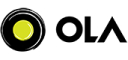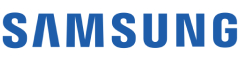New update is available. Click here to update.
Topics

# Day 17 : Count Leaf Nodes

Easy0/40
Average time to solve is 10m+3 more companies

## Problem statement

You are given a Binary tree. You have to count and return the number of leaf nodes present in it.

A binary tree is a tree data structure in which each node has at most two children, which are referred to as the left child and the right child

A node is a leaf node if both left and right child nodes of it are NULL.

Detailed explanation ( Input/output format, Notes, Images )
Constraints:
``````1 <= T <= 100
1 <= N <=10^3
1 <= data <= 10^9

Time Limit : 1 sec
``````
Sample Input 1:
``````3
1 -1 -1
1  2  3  -1 -1 -1 4 -1 -1
1  2 -1 -1 3 -1 4 -1 -1
``````
Sample Output 1:
``````1
2
1
``````
Explanation For Sample Input 1:
``````(i) 1 is the only node present in the tree, and hence it is the only leaf node.
(ii) 2 and 4 are two leaf nodes present in the tree; all other nodes have at least one child (1 has 2 and 3 and 3 has 4).
(iii) 4 is the only leaf node present in the tree as all other nodes have at least one child node (1 has 2, 2 has 3 and 3 has 4)
``````
Sample Input 2:
``````3
1 2 3 4 5 6 7 -1 -1 -1 -1 -1 -1 -1 -1
1 -1 2 -1 3 -1 -1
1 2 -1 3 4 5 6 7 8 -1 - 1 -1 -1 -1 -1 -1 -1
``````
Sample Output 2:
``````4
1
4
``````
Explanation For Sample Input 2:
``````(i) 4, 5, 6 and 7 are 4 leaf nodes present in the tree, all other nodes have at least one child (1 has 2 and 3, 2 has 4 and 5 and 3 has 6 and 7).
(ii) 3 is the only leaf node present in the tree as all other nodes have at least one child node (1 has 2 and 2 has 3)
(iii) 5, 6, 7 and 8 are 4 leaf nodes present in the tree, all other nodes have at least one child (1 has 2, 2 has 3 and 4, 3 has 5 and 6 and 4 has 7 and 8).
``````Console